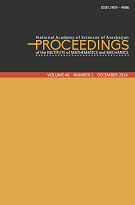P R O C E E D I N G S
of the
Institute of Mathematics and Mechanics

 ISSN 2409-4986 (Print) ISSN 2409-4994 (Online)

## Volume 44, Issue 1

Simultaneous approximation in Lebesgue space with variable exponent
by Daniyal M. Israfilov and Ahmet Testici
318
Transcendental first integrals of some classes of dynamical systems
by Maxim V. Shamolin
1935
On approximative properties of locally Chebyshev sets
by Alexey R. Alimov
3642
The inverse problem of finding the initial function for the string vibration equation
by Gunay Ismayilova
4350
Quasi-Kählerian structures carried on Codazzi connections
by Esmaeil Peyghan, Esa Sharahi and Amir Baghban
5161
Constructive method for solving the external Neumann boundary value problem for the Helmholtz equation
by Fuad A. Abdullayev and Elnur H. Khalilov
6269
On a space of $\mu$-statistical continuous functions
by Sabina R. Sadigova, Raziyya R. Hasanli and Cemil Karacam
7080
On the existence of a solution of the inverse problem for a system of parabolic equations
by Adalat Ya. Akhundov and Aynur H. Hasanova
8189
Optimization of fourth-order differential inclusions
by Elimhan N. Mahmudov
90106
Some inequalities about convolution and trigonometric approximation in weighted Orlicz spaces
by Ali Doğu, Ahmet Hamdi Avşar and Yunus Emre Yildirir
107115
Spectral properties of the problem of vibration of a loaded string in Morrey type spaces
by Telman B. Gasymov, Gular V. Maharramova and Afet N. Jabrailova
116122
Global bifurcation from infinity in some nonlinearizable eigenvalue problems with indefinite weight
by Ziyatkhan S. Aliyev and Rada A. Huseynova
123134
On strengthening of optimality conditions in discrete control systems
by Misir J. Mardanov, Telman K. Melikov and Samin T. Malik
135154
Convergence of spectral expansion of absolutely continuous vector-function in root vectors of third order differential operator with matrix coefficients
by Vali M. Kurbanov and Yulya G. Abbasova
155165
 © 2022 Institute of Mathematics and Mechanics